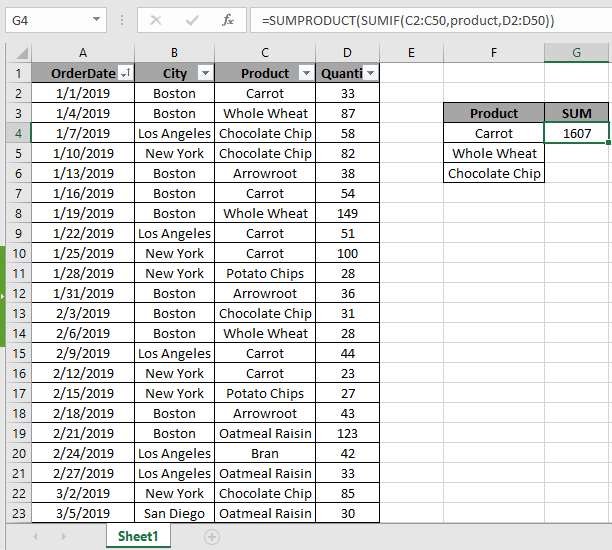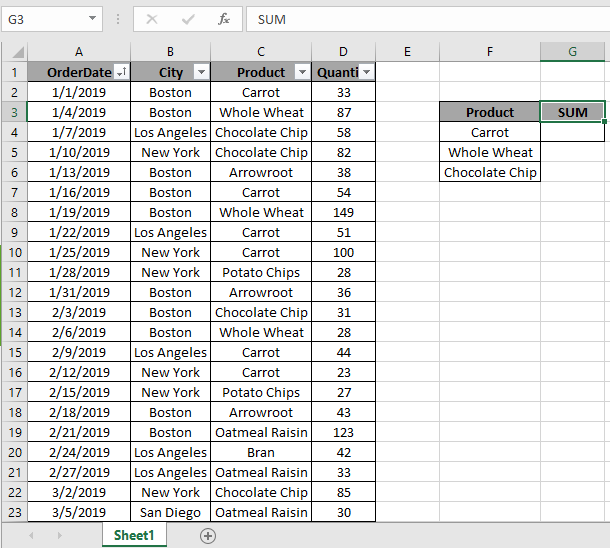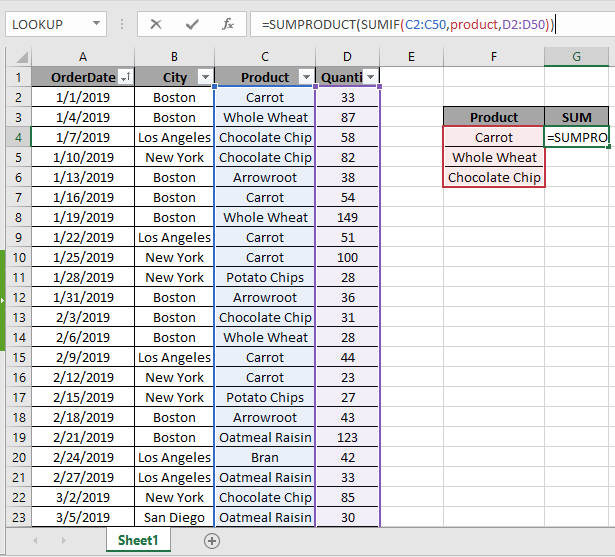# How to Sum if Equals to One of Many Things in ExcelIn this article, we will learn how to sum values when a value is equal to one of many things

In simple words, we will try sum values if any one of multiple criteria is true. The SUMIFS function works on logic. We will try to understand our logic. And to sum values with or logic, SUMPRODUCT function is always there.

How to solve the problem?

For this article we will be required to use the SUMPRODUCT function. Now we will make a formula out of these functions. Here we are given a dataset and a range and we need to match either of the values in the range and get the sum of corresponding values from dataset.

Generic formula:

= SUMPRODUCT ( SUMIF ( range, cri_range, sum_range ) )

Range: range to match with criteria range
cri_range : criteria range
sum_range : range where sum is required

Example:
All of these might be confusing to understand. So, let's test this formula via running it on the example shown below.
Here we have a dataset (A1:D50) having the order date, city, product & its quantity.Firstly, we need to find the sum of quantity where the product matches any of the values mentioned in the separate range. Now we will use the following formula to get the sum
Use the Formula:

= SUMPRODUCT ( SUMIF ( C2 : C50 , product , D2:D50 ) )

E5:E11 : range in dataset that needs to be matched.

product: criteria range

D2:D50: sum range, sum of quantity

Explanation:

• SUMIF function returns the sum if it has to match one value with the range. But here SUMIF takes the argument and returns an array to SUMPRODUCT function.

=SUMPRODUCT ( { 760 ; 348 ; 499 } )

• SUMPRODUCT function gets the returned array, which has an array of sum of quantity for different products and returns the SUM of the returned array.Here the range is given as cell reference and criteria range is given as named range. Press Enter to get the count.As you can see in the above snapshot that sum of quantity having carrot, chocolate chip or whole wheat is 1607.

Here are some observational notes shown below.

Notes:

1. The formula only works with numbers.
2. The formula only works when there are no duplicates on the lookup table
3. The SUMPRODUCT function considers non- numeric values as 0s.
4. The SUMPRODUCT function considers logic value TRUE as 1 and False as 0.
5. The argument array must be of the same length as the function.

So this is how you sum up values when any of many criteria are true. Find more articles on SUMPRODUCT functions here. Please share your query below in the comment box. We will assist you.

Related Articles

How to use the SUMPRODUCT function in Excel: Returns the SUM after multiplication of values in multiple arrays in excel.

SUM if date is between : Returns the SUM of values between the given dates or periods in excel.

Sum if date is greater than given date: Returns the SUM of values after the given date or period in excel.

2 Ways to Sum by Month in Excel: Returns the SUM of values within a given specific month in excel.

How to Sum Multiple Columns with Condition: Returns the SUM of values across multiple columns having condition in excel

How to use wildcards in excel : Count cells matching phrases using the wildcards in excel

Popular Articles

50 Excel Shortcut to Increase Your Productivity

Edit a dropdown list

Absolute reference in Excel

If with conditional formatting

Terms and Conditions of use

The applications/code on this site are distributed as is and without warranties or liability. In no event shall the owner of the copyrights, or the authors of the applications/code be liable for any loss of profit, any problems or any damage resulting from the use or evaluation of the applications/code.